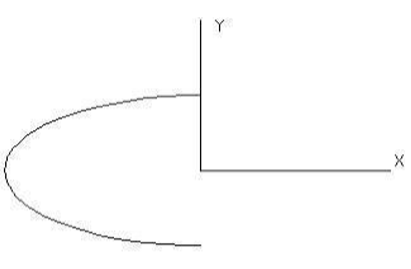# Ellipse

Conic and Ellipse tools

Conic curves or conic sections form the most general form of quadratic curves. There are eight methods for creating conics. From left to right along the Conic tool palette they are:

• Two Point Conic
• Three Point Conic
• Four Point Conic
• Center Point Ellipse
• Diagonal Ellipse
• Opposite Point Ellipse
• Three Point Ellipse
• Major Axis Ellipse

Conic

Custom Workshop Pro uses the Conic form popular in the aerospace industry. The form consists of a start and end point plus a variety of means to specify the tangency. A graphical description of the relative points is shown below.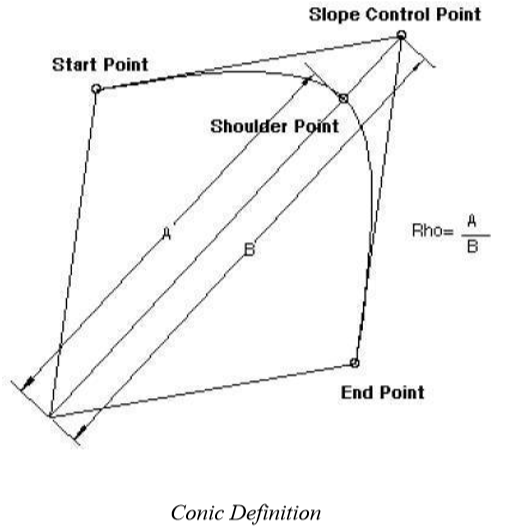Custom Workshop Pro provides three methods for creating conics that include 2, 3, and 4-point forms.

Two Point Conic

This tool creates a conic by specifying the start and endpoints plus specification of a rho value. A

rho value is the ratio of the distance of the conic center point to shoulder point, over the distance of the center point to the slope control point. See example below. This form of the conic will imply horizontal and vertical slopes relative the current construction plane for the tangencies.

Using the Two Point Conic tool

Click a point defining the conic start position. Click a point defining the conic end position. Use the Data Entry Fields to change the Rho value, if needed.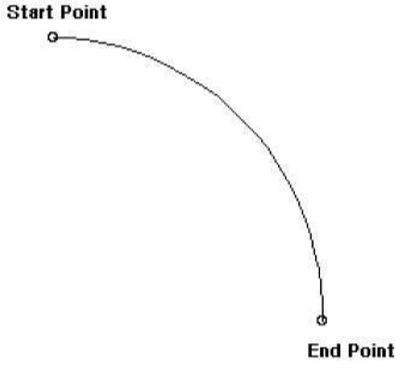Three Point Conic

Creates a conic by specifying the start, end, slope control, and the Rho value.

Using the Three Point Conic tool

Click a point defining the conic start position. Click a point defining the conic end position. Click a point defining the conic slope control position. Use the Data Entry Fields to change the rho value if needed.

Note: The slope control point is the intersection of the tangents extended from the start and end control points.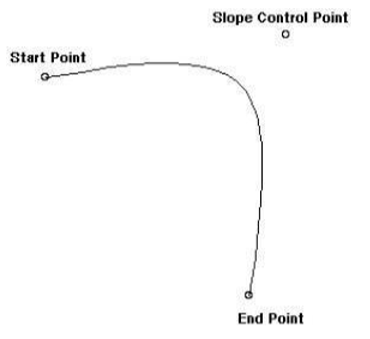Four Point Conic

Creates a conic by specifying the start point, endpoint, slope control point, and shoulder point.

Using the Four Point Conic tool

• Click a point defining the conic start position.
• Click a point defining the conic end position.
• Click a point defining the conic slope control position.
• Click a point defining the conic shoulder control position.

Note: The shoulder point lies on the conic curve.

Ellipse

Ellipses are special forms of conic curves. Ellipses in Custom Workshop Pro are defined by a center point, major axis, minor axis, and radius for each of the axes. The various user interfaces extract this information and create the appropriate ellipse. The default ellipse is created from 0 to 360 degrees, and is measured in a positive direction about the ellipse normal. The direction of the ellipse normal is defined as the cross product of the major and minor axis.

Center Point Ellipse

This command creates a 360-degree ellipse from two points that include the ellipse center and one diagonal.

Using the Center Point Ellipse tool

Click the ellipse center point.

Click the diagonal point that defines the major and minor axis lengths.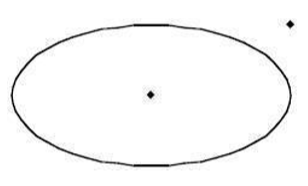Diagonal Ellipse

Creates an ellipse by opposite diagonals by indicating the upper left corner of the ellipse and the lower right.

Using the Diagonal Ellipse tool

• Click a point defining the ellipse upper left corner.
• Click a point defining the ellipse lower right corner.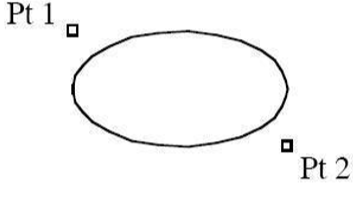Opposite Point Ellipse

Creates an ellipse by opposite ends and a point defining the height of the minor axis by indicating three points.

Using the Opposite Point Ellipse tool

• Click a point defining the ellipse left point for major axis.
• Click a point defining the ellipse right point for major axis.
• Click a point defining the ellipse height along minor axis.

Three Point Ellipse

This option creates an ellipse from three points. The first point is the ellipse center. The second point is the major axis endpoint. The third point is projected onto the minor axis to determine the correct distance.

Using the Three Point Ellipse tool

• Click a point defining the ellipse center.
• Click a point defining the ellipse major axis.
• Click a point defining the ellipse minor axis.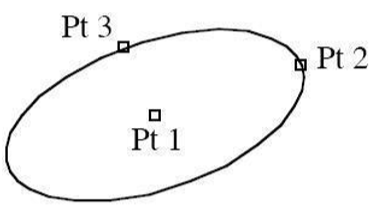Major Axis Ellipse

This option creates an ellipse by specifying two points for the magnitude and orientation of the major axis and projects a third point along the minor axis for the correct distance.

Using the Major Axis Ellipse tool

• Click a point defining the ellipse major axis start.
• Click a point defining the ellipse major axis end.
• Click a point defining the ellipse minor axis end.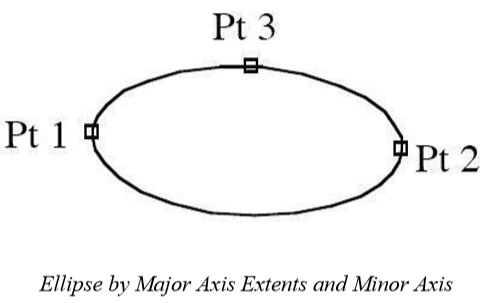Ellipse Angles

Use the Inspector to modify an ellipse’s start and end angles.